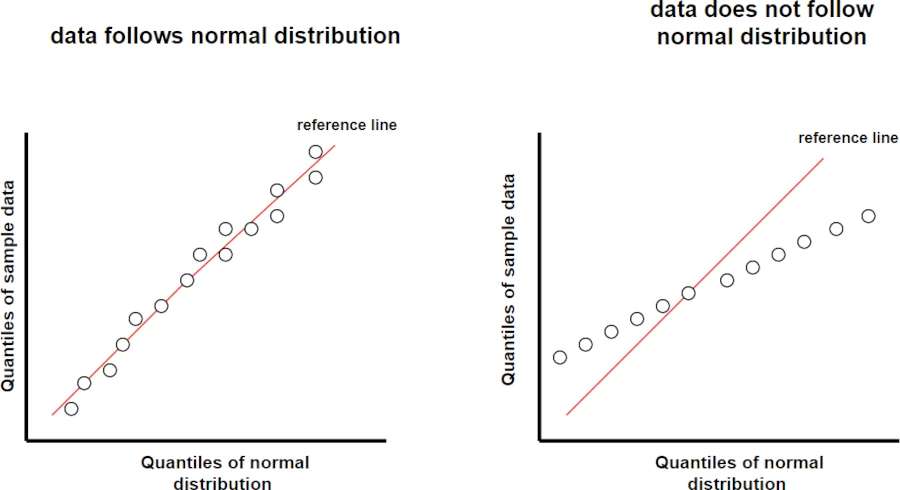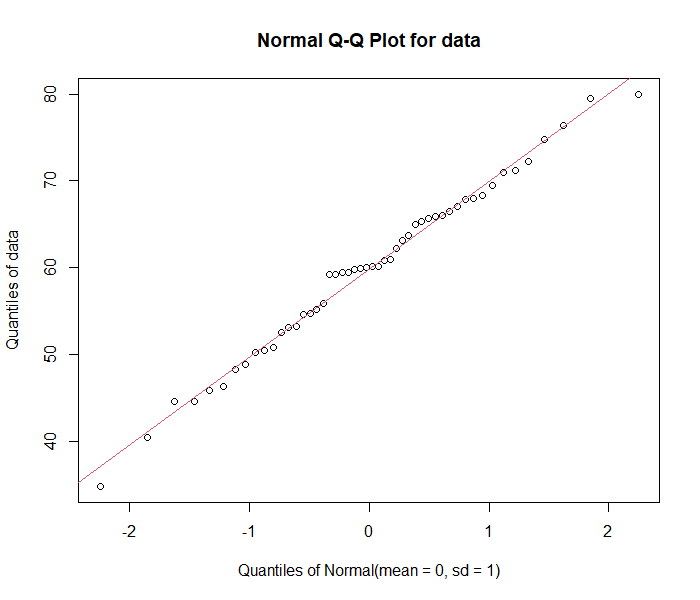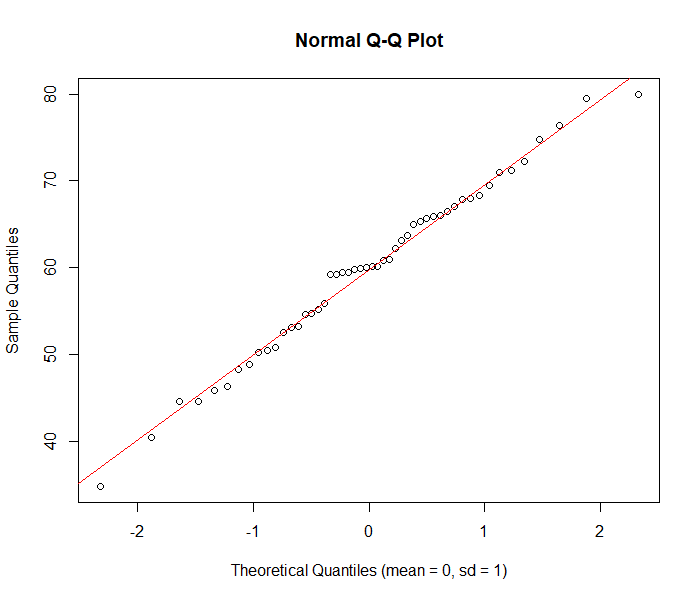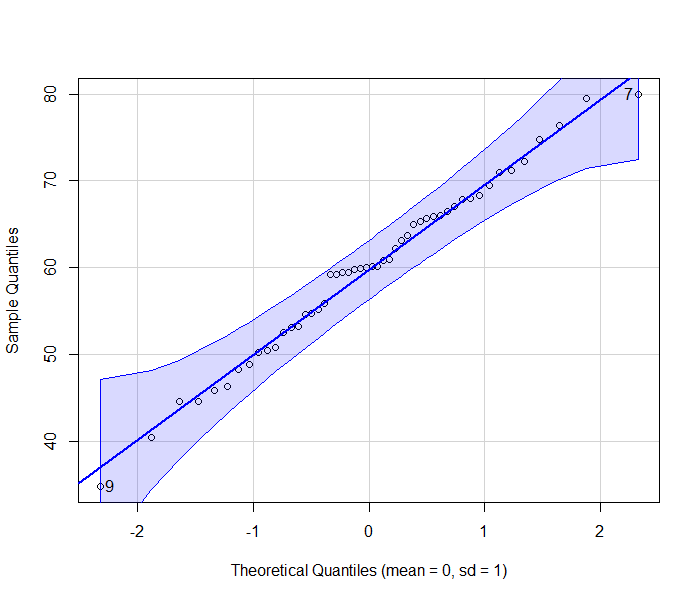# Q-Q plot in R

The Q-Q plot (Quantile-Quantile plot) is a graphical tool that compares observed quantiles of the sample data to the expected quantiles of the theoretical distribution (e.g. normal distribution).

The purpose of the Q-Q plot is to check whether the given dataset follows a normal distribution (or other theoretical distribution).

If the sample data is from normal distribution, you should see sample quantiles follows the normal quantiles in a straight line (also known as reference line).R provides a number of functions for creating QQ plots. Here’s a detailed examples on how to create a Q-Q plot in R using various functions.

## `qqPlot()` from EnvStats package

The `qqPlot()` function from EnvStats package can be used for creating Q-Q plot as below,

``````# generate random dataset with approximate normal distribution
data = rnorm(50, mean = 60, sd = 10)

# create QQ plot
library(EnvStats)

# QQ plot
qqPlot(data, add.line = TRUE, line.col = 2, line.lwd = 1.5)
``````As the observed quantiles of sample data follows the reference line, we conclude that the sample dataset follows a normal distribution.

## `qqnorm()` from base R

`qqnorm()` and `qqline()` functions can also be used for creating Q-Q plot

``````# generate random dataset with approximate normal distribution
data = rnorm(50, mean = 60, sd = 10)

# create QQ plot
qqnorm(data, xlab = "Theoretical Quantiles (mean = 0, sd = 1)")
qqline(data, col = "red", lwd = 1.5)
``````As the observed quantiles of sample data follows the reference line, we conclude that the sample dataset follows a normal distribution.

## `qqPlot()` from car package

``````# generate random dataset with approximate normal distribution
data = rnorm(50, mean = 60, sd = 10)

# create QQ plot
library(car)

# QQ plot
qqPlot(data, xlab = "Theoretical Quantiles (mean = 0, sd = 1)", ylab = "Sample Quantiles")
``````As the observed quantiles of sample data follows the reference line, we conclude that the sample dataset follows a normal distribution.

The Q-Q plot is widely used to verify the assumption of normality in hypothesis testing (e.g. ANOVA, t-test, etc.).

In addition to Q-Q plot, the assumption of normality should also be assessed using the statistical test such as Shapiro-Wilk test

## Subscribe to get new article to your email when published

* indicates required